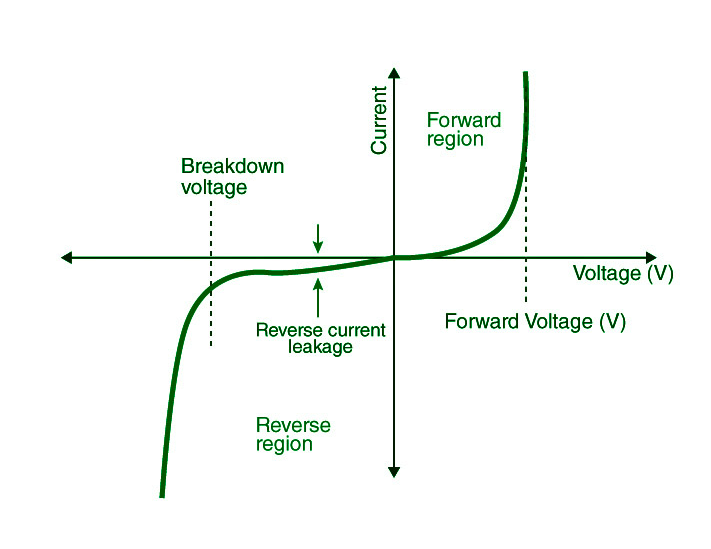# ECE492 Section 1 Notes

Welcome! Notes for Spring 2022 Electronic Circuits course. Will make this more pretty as things evolve.

My goal is to make Electronic Circuits so easily digestible, you could teach a middle schooler. We'll see if this works.

## Prologue

Hey how are you. I will insert something here later. :)

## Section 1.1

We first need to consider the following materials:

• Conductors
• Insulators
• Semiconductors

Consider the concept of the charge for Oxygen (O):

• 8 protons around the nucleus
• 8 electrons (two on the inner orbit, 6 on the outer orbit).

Let's look at the outer most orbit in context with a conductor:

• There are two bands: valency and conduction.
• Electrons are always in the valency band.
• In between, there is an energy gap, which is approximately 0 (meaning electrons can move freely between valency band and conduction band).
• That is the reason why conductive materials act as they do.

Let's look at the outer most orbit in the context of insulators:

• There are two bands: valency and conduction.
• Electrons are always in the valency band.
• In between, there is an energy gap, which is very high (meaning electrons have a very hard time moving between the valency band and conduction band).
• That is the reason insulators, are well, insulators (or their property is insulative).

Let's look at the outer most orbit in the context of semi-conductors:

• There are two bands: valency and conduction.
• Electrons are always in the valency band.
• In between, there is an energy gap, which is exactly 1.12eV (1eV = 1.602 * 10-19J).
• Once electrons are in the conduction band, the semi-conductor will act as a conductor.

This entire class is based on semi-conductor materials.

### What is a semiconductor?

It sometimes allow current, and sometimes it doesn't.

Semi-conductors only have 4 electrons in its outermost orbit.

• Carbon
• Silicon
• Germanium

What's the best two?

• Silicon and Germanium

Consideration of Silicon:

• Silicon will acquire other silicon atoms to form a covalent bond.

Now let's take a Silicon atom:

• Take a pure silicon atoms and put energy to them: go from insulator to conductive properties, and electrons from the furthest orbit detach and jump to another position.
• When electrons detach, it leaves a hole where it once was, that is viewed as a positive charge for clarity.

Doping: Adding impurity to a pure semiconductor material.

• Tri-Valent Impurity: majority of "holes" (positive charge), also know as P-type semiconductor.
• Penta-Valent Impurity: majority of electrons, also known as N-type semiconductor.

Let's take Aluminum for Tri-Valent example:

• Pair the Aluminum with Silicon atoms, knowing that Aluminum has an outer electron count of 3.
• Silicon will be bounded to the three outer Aluminum electrons, but 1 Silicon will be left.

Let's try Phosphorous for Penta-Valent example:

• Pair Phosphorous with Silicon atoms, knowing that Phosphorous has an outer electron count of 5.

Now what is P-N Junction? It's a diode.

• A P-type semiconductor connected to an N-type semiconductor.
• Recall that P-type just has a lot of "holes" (positive).
• Recall that N-type has a lot of electrons (negative).
• Shouldn't these holes and electrons combine? Electric field is present due to electrons on the N-type side of the P-N junction. This electric field does not allow for combining.
• There is an anode and a cathode on the P-type and N-type side respectively. A diode can be represented with A -> C.
• Now let's connect a battery on each side of the junction, and we supplement 0.5V.
• The electric field barrier becomes thinner and thinner, but not completely gone.
• Now apply 1.0V, the electrons and holes will push against the junction.

Now consider a reverse biased diode:

• A diode where the energy source charge is reverse from the P-N junction.
• Holes to negative and electrons to positive.

Important consideration: A P-N Junction (diode, or biased/reversed biased diode) allows current in only one direction.

What are the V-I characteristics of the P-N Junction/Diode:

• What is PIV? Peak Inverse Voltage.
• Consider the graph below for a typical V-I characteristic of a diode.Therefore, important considerations added for P-N Junction diode:

• A P-N Junction (diode, or biased/reversed biased diode) allows current in only one direction.
• Acts like a closed switch in forward biased condition.
• Acts like an open switch in reversed biased condition.

Now consider the following example: Find the current passing through each diode.

• We'll need to break the problem apart.
• Current always flows from higher potential to lower potential.
• Assume ideal diode.

Let's break the problem down:

• Step 1: Fill me in. :)

Insert example 1A

Now let's consider the following example:

• Step 1: Find all possible current flow directions (current always flows from high potential to low potential) and make a determination of whether a diode is open/closed/don't know.
• Step 2: We'll take the assumption that D2 and D3 are closed because we don't know what they are.
• Note: We can't have 3 different voltages at the same node, so our assumption is wrong.
• Step 3: Now we'll verify that our assumption is correct.
• Is D2 and D3 open? Since anode voltage is higher than cathode, then diode acts like a closed switch [in ideal case].
• Therefore, both D2 and D3 are open.

Insert example 1B

Now let's consider this next example.

• Step 1: Find I1, I2, and I3 assuming ideal diodes.
• Find all possible current flow directions (current always flows from high potential to low potential).
• Step 2: We'll assume that

### Assignment 1.1

We'll take this $x_{3}={\frac {9}{3}}$and plug it into $2x_{2}+x_{3}=5$to find x2:

{\begin{aligned}2x_{2}+x_{3}=5\\x_{3}={\frac {9}{3}}\\{\text{Plug in }}x_{3}={\frac {9}{3}}{\text{ into }}2x_{2}+x_{3}=5{\text{:}}\\2x_{2}+{\frac {9}{3}}=5\\2x_{2}=2\\x_{2}=1\end{aligned}}